Volgograd Arena - Parametric design tutorial with Revit, Dynamo & Python part 1. | DeltaCodes

# Volgograd Arena - Parametric design tutorial with Revit, Dynamo & Python part 1.

To celebrate Football WorldCup Russia 2018 we will present a series of tutorials on how to build a parametric model using Revit, Dynamo and Python scripting.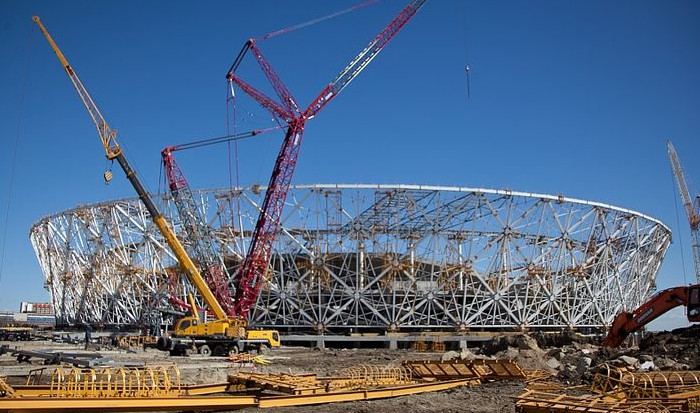We will start form constructing elevation. In a first step we will define basic shape of a stadium and create list of knot points which we will use to create structure.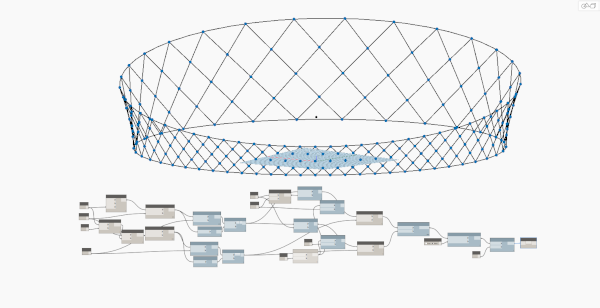The shape of Volgograd Arena is defined by to circles. The one on top has a bigger radius than this of a base. Basing on information found online we made the height of the elevation is 40 meters, diameter of upper circle 151.5 meters, base circle is smaller by ¼ of height.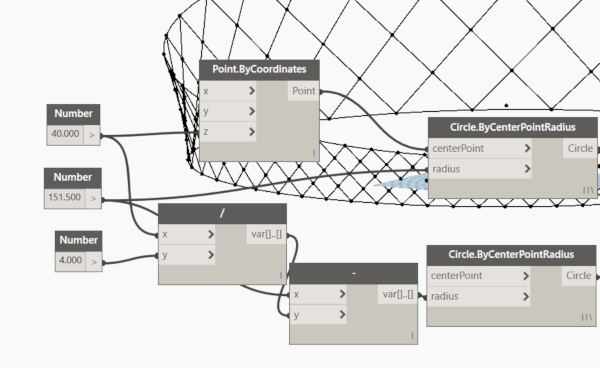There are 44 columns around the stadium so we have to divide both circles into 44 equal segments. We used Curve.PointsAtEqualChirdLength component for this. It outputs all points which are results of division so we have to add end points to the list. At the end we have to marge two lists with List.Join.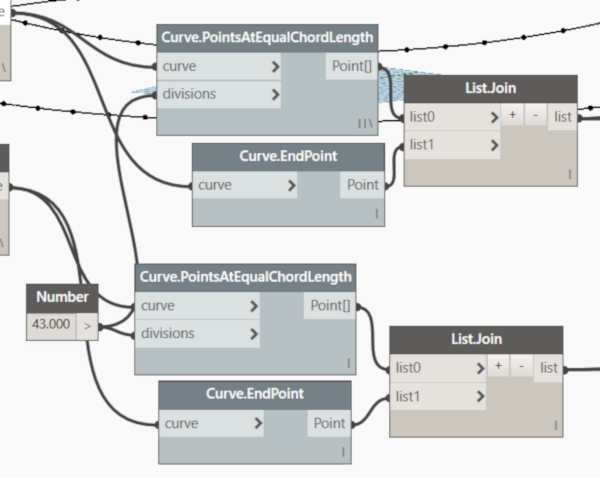To create diagonal patter of facade for have to shift list of points by to steps to the right and then to the left. Now we can connect points from the base and to shifted stet of points to create lines of this pattern...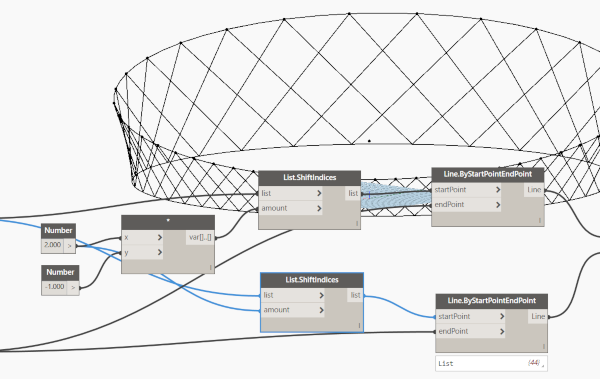The last step is to find intersection of this lines and sort list of this points by levels. For finding intersections we used Geometry.Intersect component.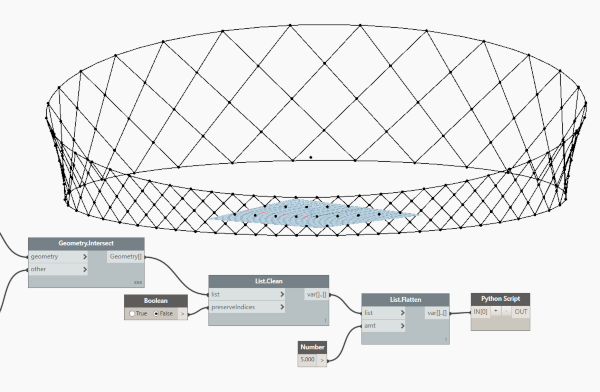Sorting list of points required a little bit of scripting. List of points was sorted by Z parameter of points, numbers were rounded to avoid errors.

`import clrclr.AddReference('ProtoGeometry')from Autodesk.DesignScript.Geometry import *#The inputs to this node will be stored as a list in the IN variables.messyListOfKnots = IN list_of_knots = []for i in range(0,5):list_of_knots.append([])for i in range(0, len(messyListOfKnots)):  if round(messyListOfKnots.Z)== round(messyListOfKnots[i].Z):   list_of_knots.append(messyListOfKnots[i])  if round(messyListOfKnots.Z) == round(messyListOfKnots[i].Z):   list_of_knots.append(messyListOfKnots[i])  if round(messyListOfKnots.Z) == round(messyListOfKnots[i].Z):   list_of_knots.append(messyListOfKnots[i])  if round(messyListOfKnots.Z) == round(messyListOfKnots[i].Z):   list_of_knots.append(messyListOfKnots[i])  if round(messyListOfKnots.Z) == round(messyListOfKnots[i].Z):   list_of_knots.append(messyListOfKnots[i])#Assign your output to the OUT variable.OUT = list_of_knots`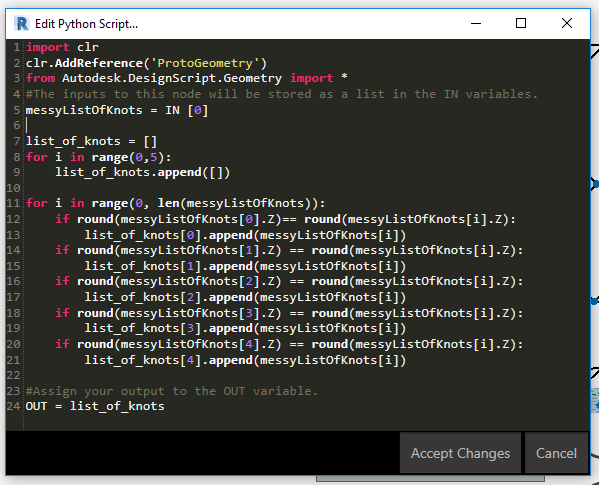Continue to second part: Volgograd Arena - Parametric design tutorial with Revit, Dynamo & Python part 2.